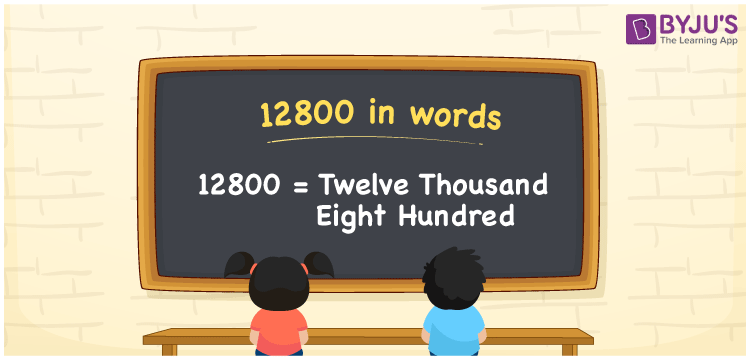# 12800 in Words

12800 in words is Twelve thousand eight hundred. We can express 12800 in words as Twelve thousand eight hundred. As we know, 12800 is a cardinal number since it represents a certain quantity. If you spent Rs. 12800 to purchase a new fitness band, you could say, “I bought a fitness band worth Twelve thousand eight hundred rupees”.

 12800 in words Twelve thousand eight hundred Twelve thousand eight hundred in numerical form 12800

## 12800 in English Words

Generally, we use English alphabets to write numbers in words and thus, we can write 12800 in English words as “Twelve thousand eight hundred”.## How to Write 12800 in Words?

The below table represents the place value chart up to 5 places since the number 12800 has five digits.

 Ten thousands Thousands Hundreds Tens Ones 1 2 8 0 0

From the above chart, we can expand the digits as:

Here, we can observe that ones = 0, tens = 0, hundreds = 8, thousands = 2, ten thousands = 1

1 × Ten thousand + 2 × Thousand + 8 × Hundred + 0 × Ten + 0 × One

= 1 × 10000 + 2 × 1000 + 8 × 100 + 0 × 10 + 0 × 1

= 10000 + 2000 + 800

= Ten Thousand + Two thousand + Eight hundred

= Twelve thousand + Eight hundred

= Twelve thousand eight hundred

12800 is a natural number that is the successor of 12799 and the predecessor of 12801.

12800 in words – Twelve thousand eight hundred

Is 12800 an odd number? – No

Is 12800 an even number? – Yes

Is 12800 a prime number? – No

Is 12800 a composite number? – Yes

Is 12800 a perfect square number? – No

Is 12800 a perfect cube number? – No

## Frequently Asked Questions on 12800 in Words

Q1

### How do you say 12800 in words?

We can spell 12800 in words as Twelve thousand eight hundred.
Q2

### How do you write Rs. 12800 on a cheque?

On a cheque, Rs. 12800 can be written as “Twelve thousand eight hundred rupees only”.
Q3

### What number is Twelve thousand eight hundred?

The number 12800 is Twelve thousand eight hundred, which means 12800 = Twelve thousand eight hundred.
Test your Knowledge on 12800 in Words